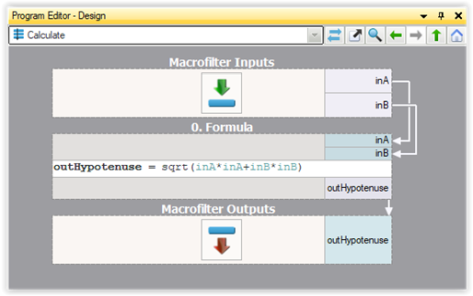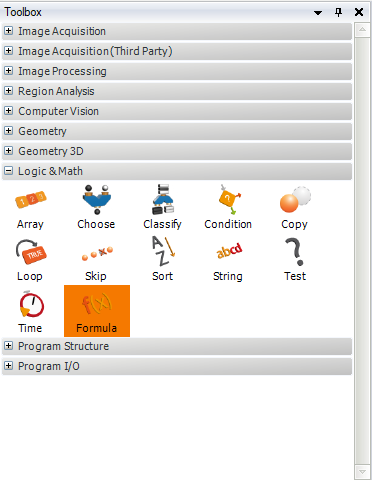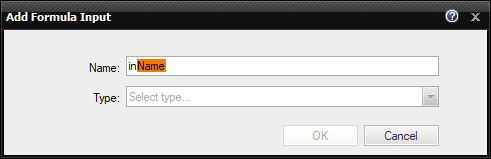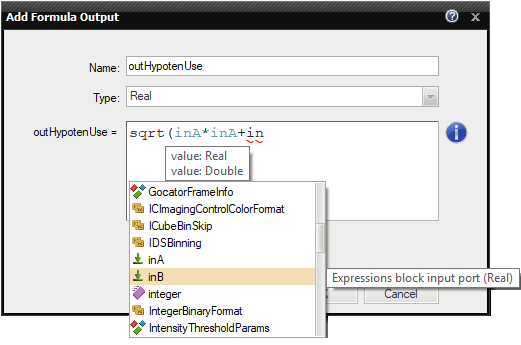You are here: Start » User Interface » Performing General Calculations

# Performing General Calculations

## Introduction

Apart from using image processing or computer vision tools, most often it is also necessary to perform some general calculations on numeric values or geometrical coordinates. There are two ways to do this in Adaptive Vision Studio:

1. With the filters of the Standard Library.
2. With special formula blocks.

## Example

Let us assume that we need to compute the hypotenuse $$\sqrt{a^2 + b^2}$$. Here are the two possible solutions:Calculations with standard filters.Calculations with formula blocks.

The second approach, with formula blocks, is the most recommended. Data flow programming is just not well suited for numerical calculations and standard formulas, that can be defined directly in formula blocks, are much more readable. You can also think about this feature as an ability to embed little spreadsheets in your machine vision algorithms.

## Creating Formula Blocks

To create a formula block:

1. Drag and drop the Formula tool from the Toolbox (Logic & Math section) to the Program Editor:3. For each input define the name and the type:4. For each output define the name, the type and the formula:Remarks

• Existing formulas can also be edited directly on a formula block in the Program Editor.
• It is also possible to create new inputs and outputs by dragging and dropping connections onto a formula block.
• Formula blocks containing incorrect formulas are marked with red background. Programs containing such filters cannot be run.
• When defining a formula for an output it is possible to use other outputs, provided that they are defined earlier. The order can be changed through the context menu of the outputs.
• For efficiency reasons it is advisable not to use "heavy" objects in formulas, such as images or regions.

## Syntax and Semantics

For complete information about the syntax and semantics please refer to the Formulas article in the Programming Reference.

 Previous: Remote Access to the Runtime Application Next: Managing Projects with Project Explorer